[中文] Search Internet Search HelloDBA
Oracle Technology Site. email: fuyuncat@gmail.com  MSN: fuyuncat@hotmail.com# Full Table Scan cost formula cracking(6)

[中文]

Author:  fuyuncat

Source:  www.HelloDBA.com

Date:  2009-08-01 15:03:32

Share to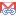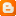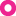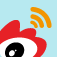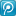### 3.4.20.OR

It become more complex after OR involved. It will at least affect 2 aspects: 1, selectivity; 2, the priority of OR & AND is different, so the process sequence will also be affected.

#### 3.4.20.1.Multiple OR on same column

This is a simple case. From the logic, it’s easily to say that multiple OR operate on same column is equal to IN. It can be proved by the test data,

 EFFQRYCOLNUM 1 FILTER COST_CPU CPU_A TYPFAC CACULATED a in (:a1, :a2) 257421440 7121440 250300000 250.3 a = :a1 or a = :a2 257421440 7121440 250300000 250.3

#### 3.4.20.2.Multiple OR on different columns

This is a complex case. The most important is that, when mixed with AND, there will be multiple priorities in the filter. Whatever, with sufficient testing, we can finally find the secret. Let’s start without AND,

 a varchar2(50), b number, c varchar2(50), d date, e date, f number,g nunber EFFQRYCOLNUM 0 a:100;b:200;c:300;d:80;e:160;f:250;g:400 TABROWS 1000000 FILTER COST_CPU CPU_A CPU_B TYPFAC b=:v2 or c=:v3 or d=:v4 366372690 7121440 359251250 359.25125 a=:v1 or b=:v2 or d=:v3 or d=:v4 415626434 7121440 408504994 408.504994 a=:v1 and b=:v2 or c=:v3 or d=:v4 366620196 7121440 359498756 359.498756 b>:v2 or c<:v3 or d>:v4 359746440 7121440 352625000 352.625 b<>:v2 or c<>:v3 or d<>:v4 267372690 7121440 260251250 260.25125 b like :v2 or c like :v3 or d like :v4 502371440 7121440 495250000 495.25

Here the rule is, the selectivity of OR become (1-Origninal Selectivity). For example, when operate OR on a IN (:a1, :a2), its selectivity become (1-2*1/100).

#### 3.4.20.3.Mix OR with AND

Then we look into mixture of OR & AND. I said before, OR & AND have different priorities, the priority of AND is higher than the OR, that is, without () limitation, it will first deal with AND then with OR. This point confused me: there are different priorities in this circumstances; the smallest processing unit may be not a single column, but a clause. For example, there are 2 priority in the filter: (a =: v1 or b <: v2) and (c =: v3 or d in (: d1,: d2)). When deal with the first priority (the 2 OR clause in parentheses), the unit is column, which can be applied the rule before. However, for the second priority, the small unit is 2 clauses. Therefore, we must first solve the problem that how to calculate COLTYPFAC and COLSEL of a clause.

 a varchar2(50), b number, c varchar2(50), d date, e date, f number,g nunber EFFQRYCOLNUM 0 a:100;b:200;c:300;d:80;e:160;f:250;g:400 TABROWS 1000000 FILTER COST_CPU CPU_A CPU_B TYPFAC b > :v2 and c = :v3 247621440 7121440 240500000 240.5 b > :v2 or c = :v3 394621440 7121440 387500000 387.5 a like :v1 and b > :v2 284621440 7121440 277500000 277.5 a like :v1 or b > :v2 419621440 7121440 412500000 412.5 a <> :v1 and b = :v2 and c in (:c1, :c2) 297956440 7121440 290835000 290.835 a <> :v1 or b = :v2 or c in (:c1, :c2) 249609773 7121440 242488333 242.488333 a like :v1 and c = :v3 and d in (:d1, :d2) 267554148 7121440 260432708 260.432708 a like :v1 and (b > :v2 or c = :v3) 306996440 7121440 299875000 299.875

Base on the test cases, I finally get the formula of them:

• AND clause

EXPTYPEFAC = MIN((COLTYPFAC1+ COLTYPFAC2*COLSEL1), (COLTYPFAC2+ COLTYPFAC1*COLSEL2)

EXPSEL = COLSEL1*COLSEL2

• OR clause

EXPTYPEFAC = MIN((COLTYPFAC1+ COLTYPFAC2*(1-COLSEL1)), (COLTYPFAC2+ COLTYPFAC1*(1-COLSEL2))

EXPSEL = COLSEL1 + COLSEL2 - COLSEL1*COLSEL2

When the unit is clause instead of column, the formula can be transformed as,

Formula 21:

• AND clause

EXPTYPEFAC = MIN((SUBEXPTYPFAC1+ SUBEXPTYPFAC2*SUBEXPSEL1), (SUBEXPTYPFAC2+ SUBEXPTYPFAC1*SUBEXPSEL2)

EXPSEL = SUBEXPSEL1*SUBEXPSEL2

• OR clause

EXPTYPEFAC = MIN((SUBEXPTYPFAC1+ SUBEXPTYPFAC2*(1-SUBEXPSEL1)), (SUBEXPTYPFAC2+ SUBEXPTYPFAC1*(1-SUBEXPSEL2))

EXPSEL = SUBEXPSEL1 + SUBEXPSEL2 - SUBEXPSEL1*SUBEXPSEL2

The SUBEXPTYPFAC and SUBEXPSEL in it could be the TYPFAC and SELECTIVITY of column or clause.

### 3.4.21.NOT

Obviously, the NOT will change the SELECTIVITY as (1-Original SELECTIVITY). From the test data, it prove this is the only impact from NOT,

 EFFQRYCOLNUM:0 not (a=:v1 and b=:v2 and c=:v3) 247293107 7121440 240171667 240.172 EFFQRYCOLNUM:1 b=:v1 327221440 7121440 320100000 320.1 EFFQRYCOLNUM:1 not (b=:v1) 347021440 7121440 339900000 339.9 EFFQRYCOLNUM:1 not (a like :v1) and not (b = :v2) 285616440 7121440 278495000 278.495 EFFQRYCOLNUM:1 (a like :v1) and (b = :v2) 284626440 7121440 277505000 277.505 EFFQRYCOLNUM:1 (a like :v1 or b = :v2) 420716440 7121440 413595000 413.595 EFFQRYCOLNUM:1 not (a like :v1 or b = :v2) 285616440 7121440 278495000 278.495 EFFQRYCOLNUM:0 (a like :v1 or b = :v2) 419621440 7121440 412500000 412.5 EFFQRYCOLNUM:0 not (a like :v1 or b = :v2) 284621440 7121440 277500000 277.5

So, the rule for NOT is it will turn back the selectivity as (1-Original SELECTIVITY), and also match the formula 22. Please note the priority of NOT is higher than AND & OR.

# 4.Conclusions

So far, the COST_CPU formula is,

Formula 22COST_CPU = TYPFAC*TABROWS + 0.32*TABBLKS*TABSIZ + 4500*TABBLKS

Among it,

TYPFAC = ROUND((130 + MAXFLTCOLPOS*20 + EXPTYPEFAC1+ EXPTYPEFAC2* EXPSEL1 + 20*EFFQRYCOLNUM*MAX(1,ROUND(EXPTYPEFAC *TABROWS,0))/TABROWS)*TABROWS,0)/TABROWS

The TYPFAC and SELECTIVITY of clause is calculated by the column/clause whose priority higher than it. The formula as below,

1.   AND clause

EXPTYPEFAC = MIN((SUBEXPTYPFAC1+ SUBEXPTYPFAC2*SUBEXPSEL1), (SUBEXPTYPFAC2+ SUBEXPTYPFAC1*SUBEXPSEL2)

EXPSEL = SUBEXPSEL1*SUBEXPSEL2

2.   OR clause

EXPTYPEFAC = MIN((SUBEXPTYPFAC1+ SUBEXPTYPFAC2*(1-SUBEXPSEL1)), (SUBEXPTYPFAC2+ SUBEXPTYPFAC1*(1-SUBEXPSEL2))

EXPSEL = SUBEXPSEL1 + SUBEXPSEL2 - SUBEXPSEL1*SUBEXPSEL2

The SUBEXPTYPFAC and SUBEXPSEL in it could be the TYPFAC and SELECTIVITY of column or clause.

TYPFAC of the data types:

 Data Type COLTYPEFAC CHAR、VARCHAR2 50 NUMBER 150 DATE 300

When operator is LIKE, COLTYPEFAC_NEW = COLTYPEFAC + 50

When operator is IN or NOT IN, COLTYPFAC_NEW = COLTYPEFAC*(1-1/NDV)^0+COLTYPEFAC*(1-1/NDV)^1+…+COLTYPEFAC*(1-1/NDV)^(INNUM-1)

Selectivity of the operators:

 Operator COLSEL >、<、<=、>= 1/20 LIKE 1/20 = 1/NDV <> 1-1/NDV IN NOTINNUM*1/NDV NOT IN (1-1/NDV)^NOTINNUM

The SELECTIVITY of NOT is (1 – Original SELECTIVITY).

The final formula we get can calculate all kinds of Full Table Scan COST with bind variables; however, there is still some constants in the formula. I did not find that these constants can be subject to the known parameters. Perhaps I did not find, or maybe they are really some constants.
To simplify the derivation, I used bind variables here. However, from the final formula, we can see it just affects the selectivity of filter columns in the use of non-bind variable. While there is document in Metalink explained how to calculate Selective (for example, A> val, selectivity is no longer 1/20, but (A_MAXVAL-val) / (A_MAXVAL-A_MINVAL)). If you are interested in non-bind variables, you put it into in the formula to verify it. In addition, there will some other factor will impact on the calculation of the cost, for example, parallel, partition and so on.

Whatever, if there is enough time, we can still be deduced other formula other than Full Table Scan. I also hope you can continue the derivation. With these formulas, I believe DBA will get more certainty when tuning the statement under CBO.

# 5.Example

To make it more understandable, I captured a real table from production, do a complex full table scan trace on it, and show the calculation process base on the formulas:

``****************``
``QUERY BLOCK TEXT``
``****************``
``select /*+ full(a) */ SI_STAT_DT_GMT, REMARKS, TO_ORDER from cs2_bkg_cfm a``
``where BKG_NUM LIKE :V1 and (MSG_ID > :v2 or BKG_STAT IN (:B1, :b2)) and not (SI_CTOFF_DT_GMT = :v3) or CNTR_AGGREGATE_STAT NOT IN (:C1, :C2, :C3)``
``*********************``
``QUERY BLOCK SIGNATURE``
``*********************``
``qb name was generated``
``signature (optimizer): qb_name=SEL\$1 nbfros=1 flg=0``
``  fro(0): flg=0 objn=64859 hint_alias="A"@"SEL\$1"``
``*****************************``
``SYSTEM STATISTICS INFORMATION``
``*****************************``
``  Using NOWORKLOAD Stats``
``  CPUSPEED: 714 millions instruction/sec``
``  IOTFRSPEED: 4096 bytes per millisecond (default is 4096)``
``  IOSEEKTIM: 10 milliseconds (default is 10)``
``***************************************``
``BASE STATISTICAL INFORMATION``
``***********************``
``Table Stats::``
``  Table: CS2_BKG_CFM  Alias:  A``
``    #Rows: 7561040  #Blks:  490172  AvgRowLen:  235.00``
``Index Stats::``
``  Index: CS2_BKG_CFM_IDX3  Col#: 2``
``    LVLS: 2  #LB: 30940  #DK: 7558140  LB/K: 1.00  DB/K: 1.00  CLUF: 7805400.00``
``… …``
``***************************************``
``SINGLE TABLE ACCESS PATH``
``  Column (#2): BKG_NUM(VARCHAR2)``
``    AvgLen: 11.00 NDV: 7558140 Nulls: 0 Density: 1.3236e-07``
``    Histogram: HtBal  #Bkts: 254  UncompBkts: 254  EndPtVals: 255``
``  Column (#6): MSG_ID(NUMBER)``
``    AvgLen: 11.00 NDV: 7561040 Nulls: 0 Density: 1.3226e-07 Min: 10948 Max: 9200001000004500480``
``    Histogram: HtBal  #Bkts: 254  UncompBkts: 254  EndPtVals: 255``
``  Column (#7): BKG_STAT(VARCHAR2)``
``    AvgLen: 10.00 NDV: 5 Nulls: 0 Density: 6.6128e-08``
``    Histogram: Freq  #Bkts: 5  UncompBkts: 378052  EndPtVals: 5``
``  Column (#18): SI_CTOFF_DT_GMT(DATE)``
``    AvgLen: 3.00 NDV: 8834 Nulls: 6252260 Density: 1.1320e-04 Min: 2415021 Max: 2455186``
``  Column (#34): CNTR_AGGREGATE_STAT(VARCHAR2)``
``    AvgLen: 15.00 NDV: 9 Nulls: 163020 Density: 0.11111``
``  Table: CS2_BKG_CFM  Alias: A``
``    Card: Original: 7561040  Rounded: 5358744  Computed: 5358744.20  Non Adjusted: 5358744.20``
``  Access Path: TableScan``
``    Cost:  134465.61  Resp: 134465.61  Degree: 0``
``      Cost_io: 132757.00  Cost_cpu: 14638940663``
``      Resp_io: 132757.00  Resp_cpu: 14638940663``
``  Best:: AccessPath: TableScan``
``         Cost: 134465.61  Degree: 1  Resp: 134465.61  Card: 5358744.20  Bytes: 0``

We can draw a tree computing table base on the priority,

 Priority Filter EXPTYPFAC EXPSEL 5 496.1136034 4 or 168.3162426 0.708733677 3 CNTR_AGGREGATE_STAT NOT IN (:C1, :C2, :C3) 133.9507716 0.702334595 3 and 115.45 0.021497566 2 not (SI_CTOFF_DT_GMT = :v3) 300 0.9998868 2 BKG_NUM LIKE :V1 100 0.05 2 OR 180 0.43 1 MSG_ID > :v2 150 0.05 1 BKG_STAT IN (:B1, :b2) 90 0.4

The final result computed by the formula is,

TYPFAC = ROUND((130+34*20+168.3162426+496.1136034)*C1176,0)/C1176 = 1474.429846

COST_CPU = 1474.429846*7561040+(4500/8192+0.32)* 490172*8192 = 14638953530.47984

The difference between the actual figure in the trace file is, (14638953530.47984 - 14638940663)/14638940663*100 = 0.000088%

And calculated by the COST formula, it’s COST is 134464.5613, which almost equal to the actual figure (134465.61).

--The end--